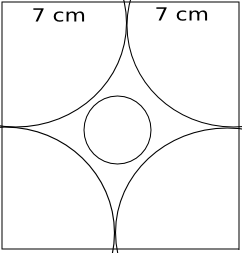Question 12

# Four horses are tethered at four corners of a square plot of side 14 m so that the adjacent horses can just reach one another. There is a small circular pond of area 20 $$m^2$$ at the centre. Find the ungrazed area.

SolutionLength of the rope tied to each horse = 7 m.
Total area of the portion that the horses can graze = 4* $$\pi 7^2$$/4 = 49$$\pi$$
Area of the circular pond = 20 $$m^2$$
So, area left ungrazed = $$14^2 - 20 - 49\pi m^2$$ = 22 $$m^2$$ (approx)